## 2015年4月7日 星期二

### 使用 CsvHelper - Part.3 其他操作說明

Github - CsvHelper
https://github.com/JoshClose/CsvHelper

CsvHelper Documentation
http://joshclose.github.io/CsvHelper/

### 判斷 CSV 檔案是否存在以及是否有資料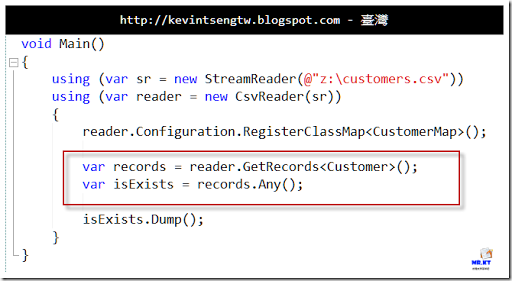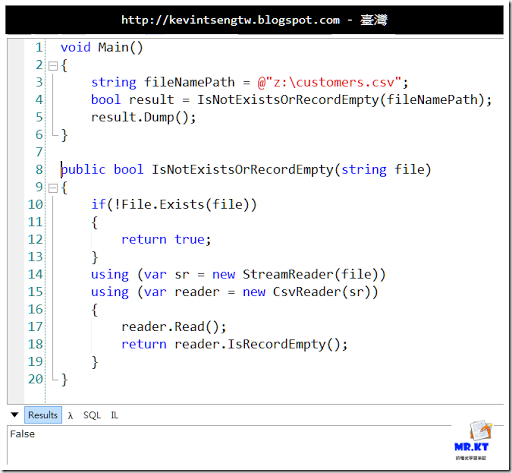### 使用 Append 方式讓資料接續寫入 CSV 檔案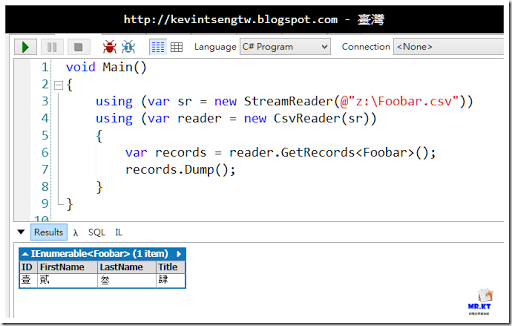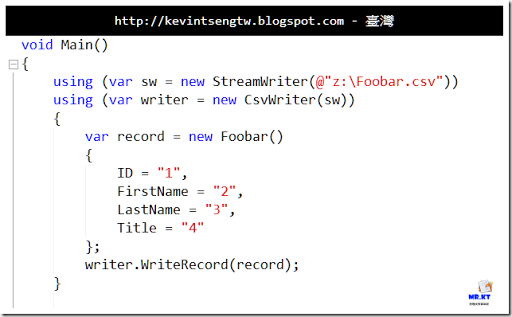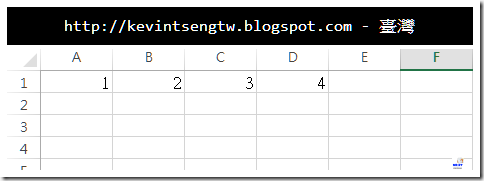“使用指定的編碼方式和預設緩衝區大小，為指定的檔案初始化 StreamWriter 類別的新執行個體。 如果檔案存在，可以將它寫入或附加。 如果檔案不存在，這個建構函式會建立新的檔案。”

“append： true 表示要附加資料至檔案，false 表示要覆寫檔案。 如果指定的檔案不存在，則這個參數沒有任何作用，而且建構函式會建立新的檔案。”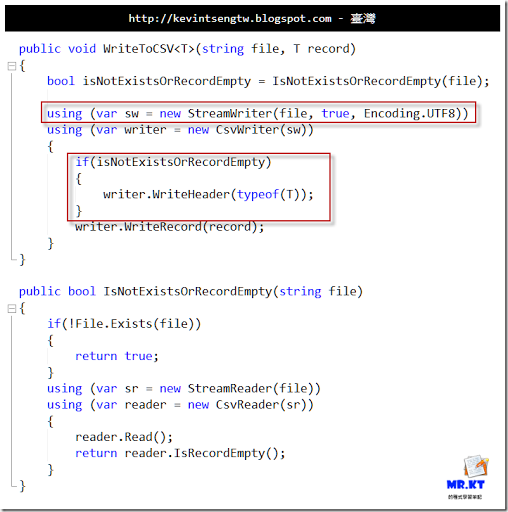`public void WriteToCSV<T>(string file, T record)`
`{`
`    bool isNotExistsOrRecordEmpty = IsNotExistsOrRecordEmpty(file);`
` `
`    using (var sw = new StreamWriter(file, true, Encoding.UTF8))`
`    using (var writer = new CsvWriter(sw))`
`    {    `
`        if(isExistsOrRecordEmpty)`
`        {`
`            writer.WriteHeader(typeof(T));`
`        }`
`        writer.WriteRecord(record);`
`    }`
`}`
` `
`public bool IsNotExistsOrRecordEmpty(string file)`
`{`
`    if(!File.Exists(file))`
`    {`
`        return true;`
`    }`
`    using (var sr = new StreamReader(file))`
`    using (var reader = new CsvReader(sr))`
`    {`
`        reader.Read();`
`        return reader.IsRecordEmpty();`
`    }    `
`}`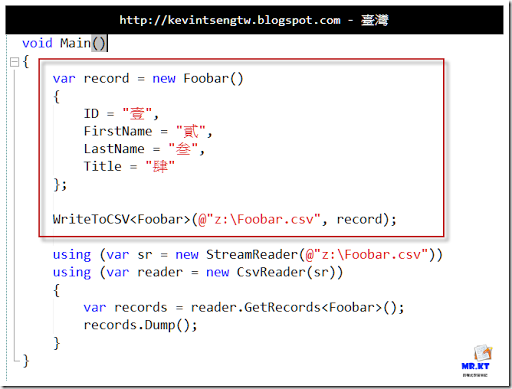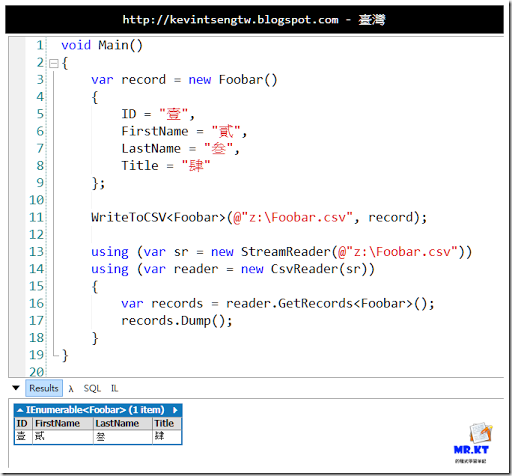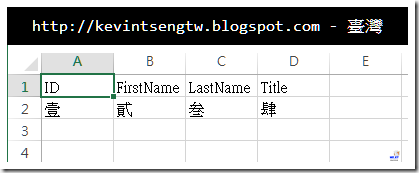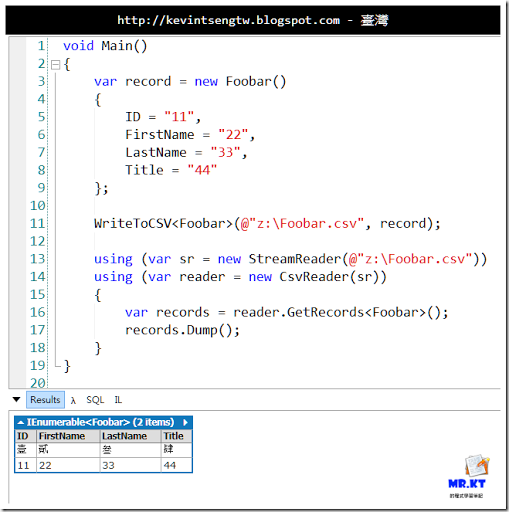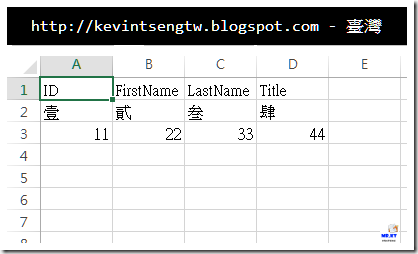`void Main()`
`{`
`    string fileName = @"z:\Foobar.csv";`
` `
`    var record = new Foobar()`
`    { `
`        ID = "111", `
`        FirstName = "222", `
`        LastName = "333", `
`        Title = "444" `
`    };`
`    `
`    WriteToCSV<Foobar>(fileName, record);    `
`    var records = ReadFromCSV<Foobar>(fileName);`
`    records.Dump();`
`}`
` `
`public IEnumerable<T> ReadFromCSV<T>(string file)`
`{`
`    using (var sr = new StreamReader(file))`
`    using (var reader = new CsvReader(sr))`
`    {        `
`        var records = reader.GetRecords<T>();        `
`        return records.ToList();`
`    }`
`}`
` `
`public void WriteToCSV<T>(string file, T record)`
`{`
`    bool isNotExistsOrRecordEmpty = IsNotExistsOrRecordEmpty(file);`
` `
`    using (var sw = new StreamWriter(file, true, Encoding.UTF8))`
`    using (var writer = new CsvWriter(sw))`
`    {    `
`        if(isNotExistsOrRecordEmpty)`
`        {`
`            writer.WriteHeader(typeof(T));`
`        }`
`        writer.WriteRecord(record);`
`    }`
`}`
` `
`public bool IsNotExistsOrRecordEmpty(string file)`
`{`
`    if(!File.Exists(file))`
`    {`
`        return true;`
`    }`
`    using (var sr = new StreamReader(file))`
`    using (var reader = new CsvReader(sr))`
`    {`
`        reader.Read();`
`        return reader.IsRecordEmpty();`
`    }    `
`}`
` `
`public class Foobar`
`{`
`    public string ID { get; set; }`
`    `
`    public string FirstName { get; set; }`
`    `
`    public string LastName { get; set; }`
`    `
`    public string Title { get; set; }`
`}`